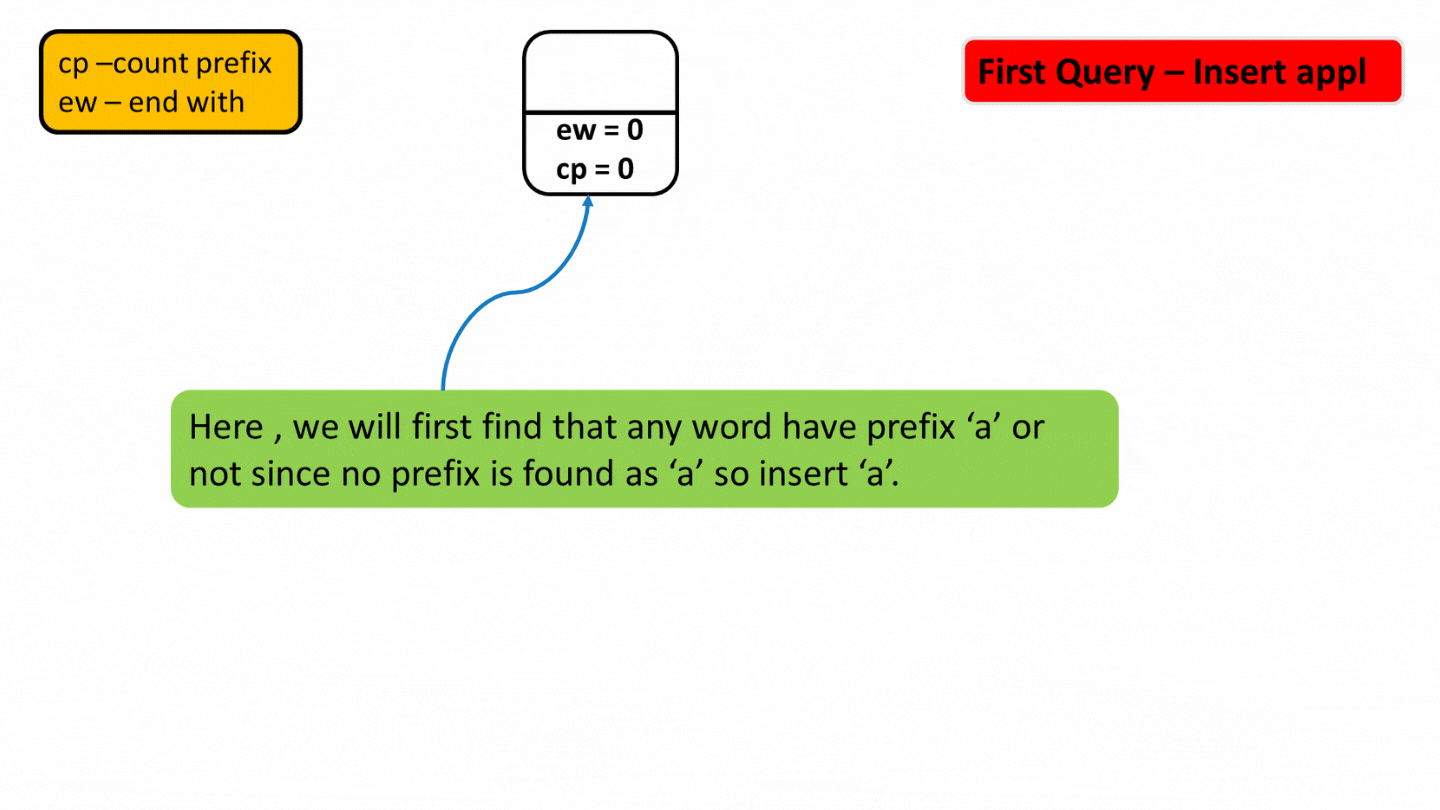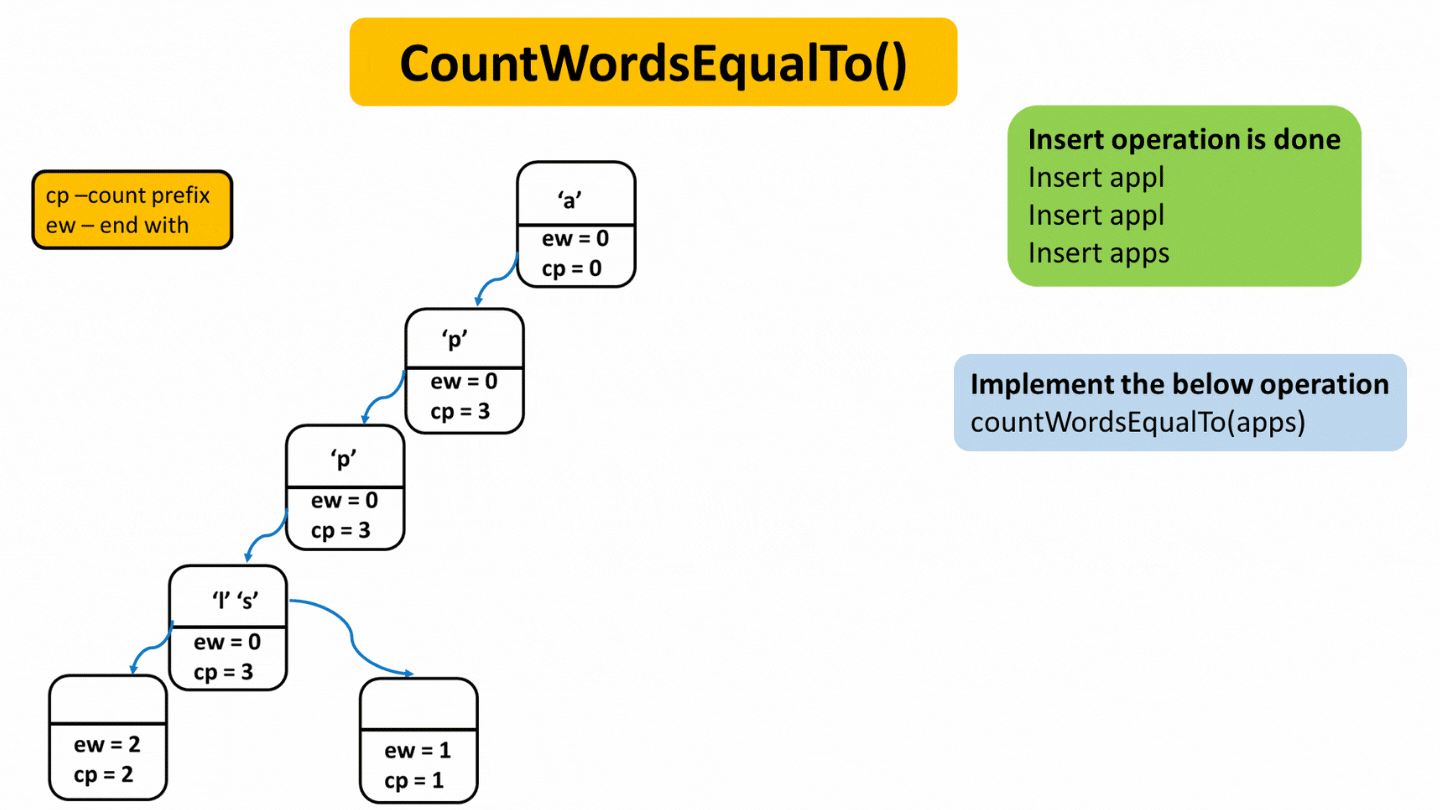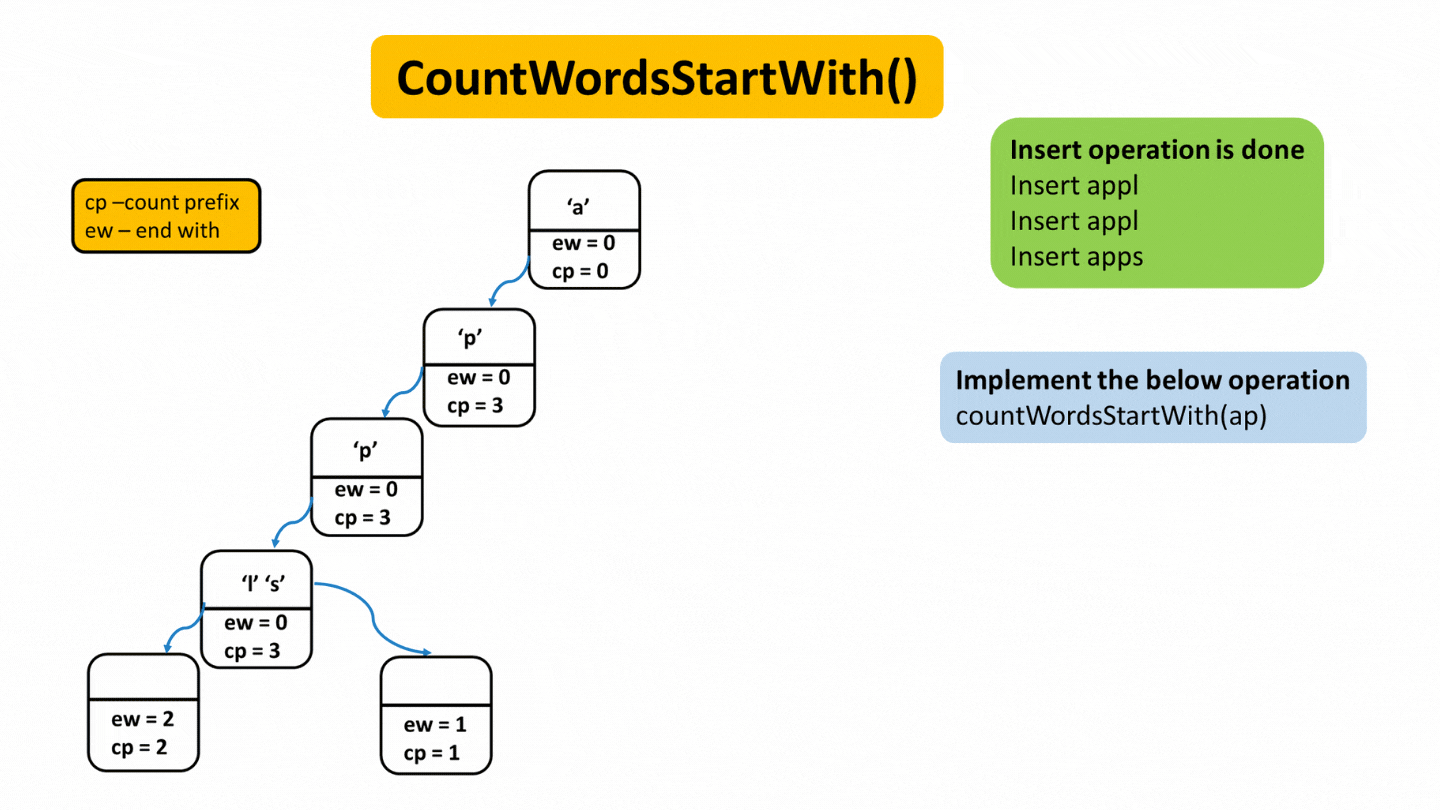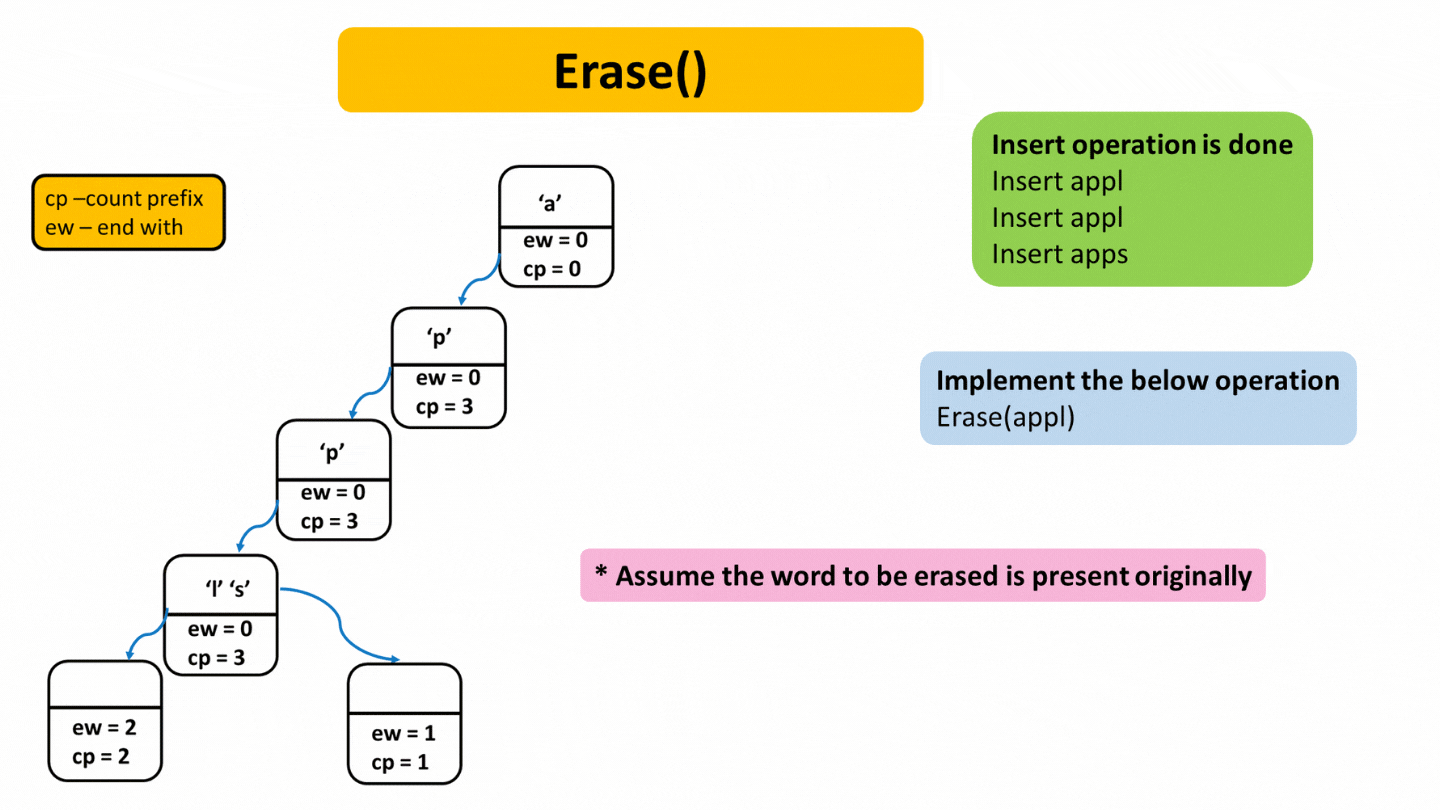# Implement Trie – II

Problem Statement:  Implement a data structure ”TRIE” from scratch. Complete some functions.

1) Trie(): Initialize the object of this “TRIE” data structure.

2) insert(“WORD”): Insert the string “WORD”  into this “TRIE” data structure.

3) countWordsEqualTo(“WORD”): Return how many times this “WORD” is present in this “TRIE”.

4) countWordsStartingWith(“PREFIX”): Return how many words are there in this “TRIE” that have the string “PREFIX” as a prefix.

5) erase(“WORD”): Delete this string “WORD” from the “TRIE”.

Note:

1. If erase(“WORD”) function is called then it is guaranteed that the “WORD” is present in the “TRIE”.

2. If you are going to use variables with dynamic memory allocation then you need to release the memory associated with them at the end of your solution.

```Example:
Input:
1
6
insert samsung
insert samsung
insert  vivo
erase vivo
countWordsEqualTo samsung
countWordsStartingWith vi
Output:
2
0

Explanation:

Insert “samsung”: we are going to insert the word “samsung” into the “TRIE”.
Insert “samsung”: we are going to insert the word “samsung” again into the “TRIE”.
Insert “vivo”: we are going to insert the word “vivo” into the “TRIE”.
Erase “vivo”: we are going to delete the word “vivo” from the “TRIE”.
countWordsEqualTo “samsung”: There are two instances of “samsung” is present in “TRIE”.
countWordsStartWith “vi”:There is not a single word in the “TRIE” that starts from the prefix “vi”.
```

### Solution

Disclaimer: Don’t jump directly to the solution, try it out yourself first.

Approach

1. Insert

Here we will maintain ew -> end with  and  cp -> count prefix,
We will insert letter by letter by creating a new track every time. Starting from the root, fill letters of the word one by one and subsequently increase cp. At the end of every word, increase ew which denotes that word finished here. At the time a different prefix is found, we will create a new track by increasing cp.2. countwordsEqualTo() – This function will count complete words which are present in the Trie. Starting from the root, we will check for the given letter of the word to be found, if we find that cp >0 every time and at the end if (ew>0) this means word ends here means the complete word is present here.3. countWordsStartWith() – This function will count words starting with given string. Similarly, here also we begin from the root and check for the given letter one by one and check for cp>0. If it is, this means the given words are present here.4. Erase() – It will delete the string given from the Trie.

Note- In Erase() function, it is assumed that the given letter to be erased is present in the trie.

Starting from the root, cp for the given prefix element is greater than 0, reduce cp by 1 and move on to a new track, and at last, reduce ew by 1  to mark that. word is fully deleted.Code:

## C++ Code

``````#include <bits/stdc++.h>

using namespace std;
struct Node {
int cntEndWith = 0;
int cntPrefix = 0;

bool containsKey(char ch) {
return (links[ch - 'a'] != NULL);
}
Node * get(char ch) {
return links[ch - 'a'];
}
void put(char ch, Node * node) {
links[ch - 'a'] = node;
}
void increaseEnd() {
cntEndWith++;
}
void increasePrefix() {
cntPrefix++;
}
void deleteEnd() {
cntEndWith--;
}
void reducePrefix() {
cntPrefix--;
}
int getEnd() {
return cntEndWith;
}
int getPrefix() {
return cntPrefix;
}
};
class Trie {
private:
Node * root;

public:
/** Initialize your data structure here. */
Trie() {
root = new Node();
}

/** Inserts a word into the trie. */
void insert(string word) {
Node * node = root;
for (int i = 0; i < word.length(); i++) {
if (!node -> containsKey(word[i])) {
node -> put(word[i], new Node());
}
node = node -> get(word[i]);
node -> increasePrefix();
}
node -> increaseEnd();
}

int countWordsEqualTo(string &word)
{
Node *node = root;
for (int i = 0; i < word.length(); i++)
{
if (node->containsKey(word[i]))
{
node = node->get(word[i]);
}
else
{
return 0;
}
}
return node->getEnd();
}

int countWordsStartingWith(string & word) {
Node * node = root;
for (int i = 0; i < word.length(); i++) {
if (node -> containsKey(word[i])) {
node = node -> get(word[i]);
} else {
return 0;
}
}
return node -> getPrefix();
}

void erase(string & word) {
Node * node = root;
for (int i = 0; i < word.length(); i++) {
if (node -> containsKey(word[i])) {
node = node -> get(word[i]);
node -> reducePrefix();
} else {
return;
}
}
node -> deleteEnd();
}
};

int main() {
Trie T;
T.insert("apple");
T.insert("apple");
T.insert("apps");
T.insert("apps");
string word1 = "apps";
cout<<"Count Words Equal to "<< word1<<" "<<T.countWordsEqualTo(word1)<<endl;
string word2 = "abc";
cout<<"Count Words Equal to "<< word2<<" "<<T.countWordsEqualTo(word2)<<endl;
string word3 = "ap";
cout<<"Count Words Starting With "<<word3<<" "<<T.countWordsStartingWith(word3)
<<endl;
string word4 = "appl";
cout<<"Count Words Starting With "<< word4<<" "<<T.countWordsStartingWith(word4)
<<endl;
T.erase(word1);
cout<<"Count Words equal to "<< word1<<" "<<T.countWordsEqualTo(word1)<<endl;
return 0;
}``````

Output:

Count Words Ending With apps 2
Count Words Ending With abc 0
Count Words Starting With ap 4
Count Words Starting With appl 2
Count Words Ending With apps 1

Time Complexity: O(N), where n denotes the length of the longest string that we are inserting.

Space Complexity: O(1), since constant size array created for links.

## Java Code

``````import java.util.*;
class Node {
Node links[] = new Node;
int cntEndWith = 0;
int cntPrefix = 0;

public Node() {
}

boolean containsKey(char ch) {
return (links[ch - 'a'] != null);
}
Node get(char ch) {
}
void put(char ch, Node node) {

}
void increaseEnd() {
cntEndWith++;
}
void increasePrefix() {
cntPrefix++;
}
void deleteEnd() {
cntEndWith--;
}
void reducePrefix() {
cntPrefix--;
}
int getEnd() {
return cntEndWith;
}
int getPrefix() {
return cntPrefix;
}
}
public class Trie {
private Node root;

//Initialize your data structure here

Trie() {
root = new Node();
}

//Inserts a word into the trie

public void insert(String word) {
Node node = root;
for(int i = 0;i<word.length();i++) {
if(!node.containsKey(word.charAt(i))) {
node.put(word.charAt(i), new Node());
}
node = node.get(word.charAt(i));
node.increasePrefix();
}
node.increaseEnd();
}

public int countWordsEqualTo(String word) {
Node node = root;
for(int i = 0;i<word.length();i++) {
if(node.containsKey(word.charAt(i))) {
node = node.get(word.charAt(i));
}
else {
return 0;
}
}
return node.getEnd();
}

public int countWordsStartingWith(String word) {
Node node = root;
for(int i = 0;i<word.length();i++) {
if(node.containsKey(word.charAt(i))) {
node = node.get(word.charAt(i));
}
else {
return 0;
}
}
return node.getPrefix();
}

public void erase(String word) {
Node node = root;
for(int i = 0;i<word.length();i++) {
if(node.containsKey(word.charAt(i))) {
node = node.get(word.charAt(i));
node.reducePrefix();
}
else {
return;
}
}
node.deleteEnd();
}
public static void main(String args[]) {
Trie T=new Trie();
T.insert("apple");
T.insert("apple");
T.insert("apps");
T.insert("apps");
String word1 = "apps";
System.out.println("Count Words Equal to "+word1+" "+T.countWordsEqualTo(word1));
String word2 = "abc";
System.out.println("Count Words Equal to "+word2+" "+T.countWordsEqualTo(word2));
String word3 = "ap";
System.out.println("Count Words Starting With "+word3+" "+T.countWordsStartingWith
(word3));
String word4 = "appl";
System.out.println("Count Words Starting With "+word4+" "+T.countWordsStartingWith
(word4));
T.erase(word1);
System.out.println("Count Words equal to "+word1+" "+T.countWordsEqualTo(word1));

}

}``````

Output:

Count Words Ending With apps 2
Count Words Ending With abc 0
Count Words Starting With ap 4
Count Words Starting With appl 2
Count Words Ending With apps 1

Time Complexity: O(N), where n denotes the length of the longest string that we are inserting.

Space Complexity: O(1), since constant size array created for links.

Special thanks to Gurmeet Singh for contributing to this article on takeUforward. If you also wish to share your knowledge with the takeUforward fam, please check out this article